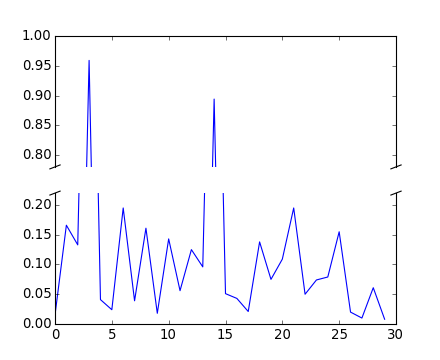#### Previous topic

pylab_examples example code: break.py

#### Next topic

pylab_examples example code: broken_barh.py

# pylab_examples example code: broken_axis.py¶```"""
Broken axis example, where the y-axis will have a portion cut out.
"""
import matplotlib.pylab as plt
import numpy as np

# 30 points between 0 0.2] originally made using np.random.rand(30)*.2
pts = np.array([ 0.015,  0.166,  0.133,  0.159,  0.041,  0.024,  0.195,
0.039, 0.161,  0.018,  0.143,  0.056,  0.125,  0.096,  0.094, 0.051,
0.043,  0.021,  0.138,  0.075,  0.109,  0.195,  0.05 , 0.074, 0.079,
0.155,  0.02 ,  0.01 ,  0.061,  0.008])

# Now let's make two outlier points which are far away from everything.
pts[[3,14]] += .8

# If we were to simply plot pts, we'd lose most of the interesting
# details due to the outliers. So let's 'break' or 'cut-out' the y-axis
# into two portions - use the top (ax) for the outliers, and the bottom
# (ax2) for the details of the majority of our data
f,(ax,ax2) = plt.subplots(2,1,sharex=True)

# plot the same data on both axes
ax.plot(pts)
ax2.plot(pts)

# zoom-in / limit the view to different portions of the data
ax.set_ylim(.78,1.) # outliers only
ax2.set_ylim(0,.22) # most of the data

# hide the spines between ax and ax2
ax.spines['bottom'].set_visible(False)
ax2.spines['top'].set_visible(False)
ax.xaxis.tick_top()
ax.tick_params(labeltop='off') # don't put tick labels at the top
ax2.xaxis.tick_bottom()

# This looks pretty good, and was fairly painless, but you can get that
# cut-out diagonal lines look with just a bit more work. The important
# thing to know here is that in axes coordinates, which are always
# between 0-1, spine endpoints are at these locations (0,0), (0,1),
# (1,0), and (1,1).  Thus, we just need to put the diagonals in the
# appropriate corners of each of our axes, and so long as we use the
# right transform and disable clipping.

d = .015 # how big to make the diagonal lines in axes coordinates
# arguments to pass plot, just so we don't keep repeating them
kwargs = dict(transform=ax.transAxes, color='k', clip_on=False)
ax.plot((-d,+d),(-d,+d), **kwargs)      # top-left diagonal
ax.plot((1-d,1+d),(-d,+d), **kwargs)    # top-right diagonal

kwargs.update(transform=ax2.transAxes)  # switch to the bottom axes
ax2.plot((-d,+d),(1-d,1+d), **kwargs)   # bottom-left diagonal
ax2.plot((1-d,1+d),(1-d,1+d), **kwargs) # bottom-right diagonal